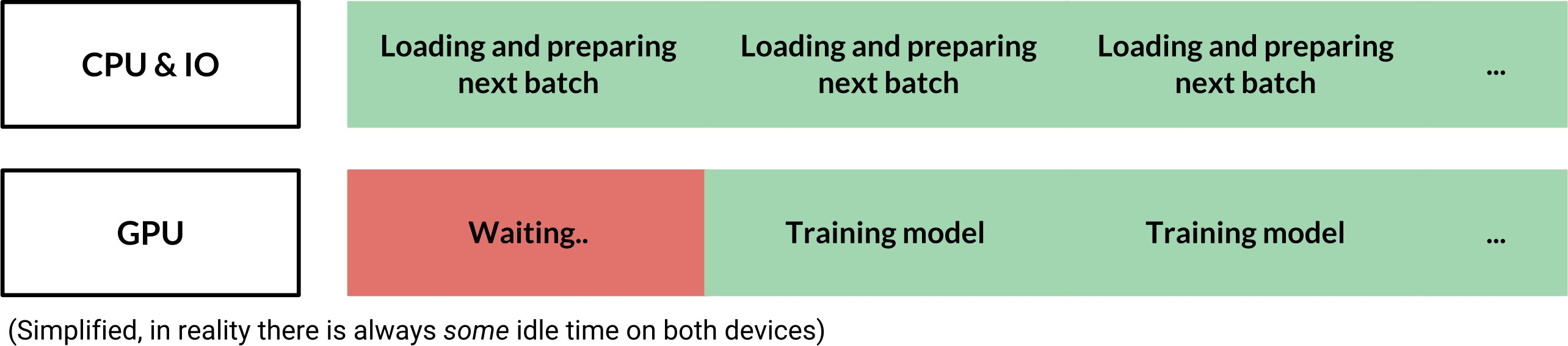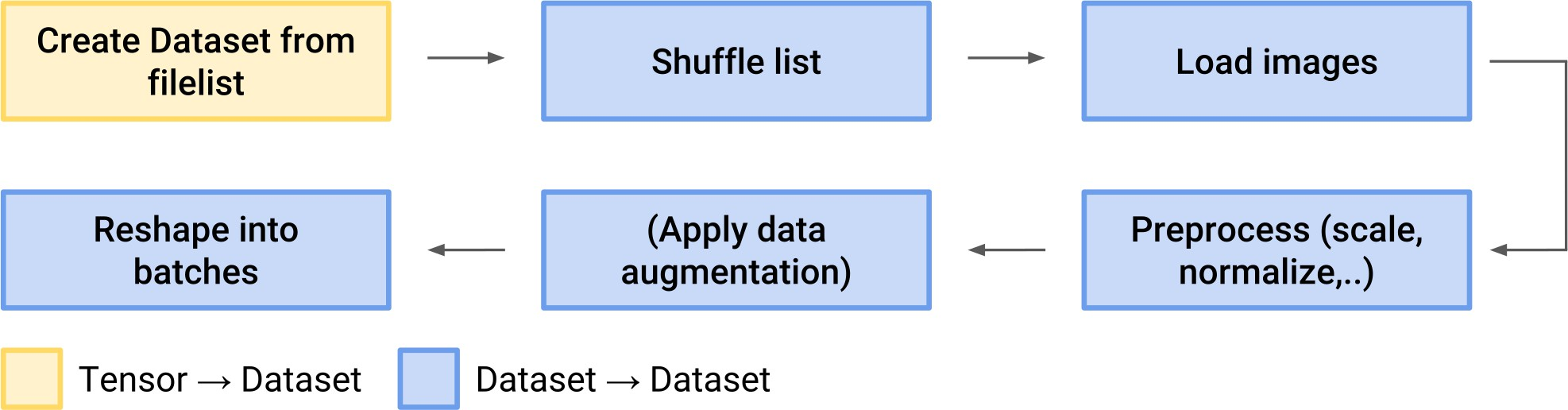## 1. feed_dict 和 tf.data 对比

Tensorflow 入门手册中一般介绍的是采用 feed_dict方法，在tf.Seession.run() 会话运行或 tf.Tensor.eval() 函数调用时，将数据加载进模型. 然而，还有另一种更加有效和更简单的方式，即，采用 tf.data API，只需几行代码即可实现高效的数据管道(pipelines).

feed_dict 管道中，GPU 存在等待时间，需要等 CPU 提供下一个 batch 的数据. 如图：## 2. 图像数据管道简单构建

• tf.data.Dataset - 保存数据；
• tf.data.Iterator - 用于从数据集中逐个提取数据样本.

[
[Tensor(image), Tensor(label)],
[Tensor(image), Tensor(label)],
...
]

• Dataset(图片文件列表) → Dataset (真实图像数据)
• Dataset(6400 images) → Dataset(64 batches with 100 images each)
• Dataset(音频文件列表) → Dataset(打乱的音频文件列表)

## 3. 定义计算图# 定义文件列表
files = ['a.png', 'b.png', 'c.png', 'd.png']

# 从文件名创建数据集
dataset = tf.data.Dataset.from_tensor_slices(files)

# 定义从路径加载图像的函数(作为张量).
# 并采用 tf.data.Dataset.map() 函数，用于数据集中的所有文件(文件路径).
# 还可以采用 map() 的 num_parallel_calls=n 参数，以并行化函数调用.

# Don't use tf.image.decode_image, or the output shape will be undefined
image = tf.image.decode_jpeg(image_string, channels=3)

# This will convert to float values in [0, 1]
image = tf.image.convert_image_dtype(image, tf.float32)

image = tf.image.resize_images(image, [image_size, image_size])
return image

# Apply the function load_image to each filename in the dataset

# 采用 tf.data.Dataset.batch() 创建 batches 数据.
# Create batches of 64 images each
dataset = dataset.batch(64)

tf.data.Dataset.prefetch(buffer_size)

dataset = dataset.prefetch(buffer_size=1)

iterator = dataset.make_initializable_iterator()

batch_of_images = iterator.get_next()

## 4. 运行会话

with tf.Session() as session:

for i in range(epochs):
session.run(iterator.initializer)

try:
# Go through the entire dataset
while True:
image_batch = session.run(batch_of_images)

except tf.errors.OutOfRangeError:
print('End of Epoch.')

nvidia-smi 命令用于监视 GPU 的利用率使用情况，有助于理解数据管道的瓶颈. 平均 GPU 利用率应该多大于 70-80%.

## 5. 数据管道构建的复杂版本### 5.1 Shuffle

dataset = tf.data.Dataset.from_tensor_slices(files)
dataset = dataset.shuffle(len(files))

### 5.2 Data Augmentation

def train_preprocess(image):
image = tf.image.random_flip_left_right(image)

image = tf.image.random_brightness(image, max_delta=32.0 / 255.0)
image = tf.image.random_saturation(image, lower=0.5, upper=1.5)
# Make sure the image is still in [0, 1]
image = tf.clip_by_value(image, 0.0, 1.0)

return image

### 5.3 Labels

# files is a python list of image filenames
# labels is a numpy array with label data for each image
dataset = tf.data.Dataset.from_tensor_slices((files, labels))

def load_image(path, label):
dataset = dataset.map(load_image)